# Class 12 Maths Chapter 9 NCERT Solutions Differential Equations

In this page we have NCERT Solutions for Class 12 Maths Chapter 9: Differential Equations for EXERCISE 9.2 . Hope you like them and do not forget to like , social share and comment at the end of the page.
In each of the Exercises 1 to 10 verify that the given functions (explicit or implicit) is a solution of the corresponding differential equation
Question 1: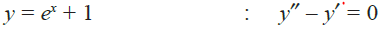Solution
y= ex + 1
Differentiating both sides of this equation with respect to x, we get:
y = ex
Now, differentiating equation (1) with respect to x, we get:
y’’ =ex
Substituting the values of in the given differential equation, we get the L.H.S.
as:
ex  -ex =0
Thus, the given function is the solution of the corresponding differential equation.
Question 2:
y=x2 +2x+C     :  (dy/dx)  -2x -2 =0
Solution
Differentiating both sides of this equation with respect to x, we get:
(dy/dx) =  2x +2
Substituting the value  in the given differential equation, we get:
L.H.S. =
(dy/dx)  -2x -2 =0
=(2x+2) -2x-2=0
= R.H.S.
So, the given function is the solution of the corresponding differential equation.

Question 3:
y= cos x + C   :       (dy/dx) +sin x =0
Solution
Differentiating both sides of this equation with respect to x, we get:
(dy/dx) = -sin x
Substituting the value in the given differential equation, we get:
L.H.S. =
(dy/dx) +sin x
=-sin x + sin x
=0
= R.H.S.
So, the given function is the solution of the corresponding differential equation.
Question 4: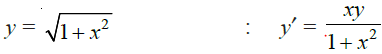Solution
Differentiating both sides of the equation with respect to x, we get: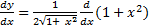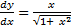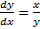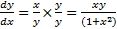So, the given function is the solution of the corresponding differential equation.

Question 5:
y = Ax :    x(dy/dx)  = y    (x≠0)
Solution
Differentiating both sides with respect to x, we get:
dy/dx = A
Substituting the value of in the given differential equation, we get:
LHS
=x(dy/dx)
=Ax
=y
=RHS
So, the given function is the solution of the corresponding differential equation.
Question 6: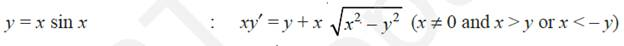Solution
Differentiating both sides of this equation with respect to x, we get:
dy/dx = sin x + x cos x
Substituting the value  in the given differential equation, we get:
LHS
=x(dy/dx)
= x(sin x + x cos x)
=x sin (x) + x2 cos (x)   -(1)
Now we know that
cos2 x = 1 – sin2 x
cos (x) = √( 1 – sin2 x)
Now we know that
y=x sin x  or sin (x) = y/x
Therefore
cos (x) = √[ 1 –(y/x)2 ]   - (2)
Substituting the value from  (2) in (1)
=x sin (x) + x2 √[ 1 –(y/x)2 ]
= y + x √(x2 – y2)
=RHS
Hence, the given function is the solution of the corresponding differential equation.
Question 7:
xy= log y + c     :  dy/dx = y2 /( 1-xy) ,  xy  ≠0
Solution
Differentiating both sides of this equation with respect to x, we get:
y +x (dy/dx) = (1/y) (dy/dx)
y2 + xy (dy/dx) = (dy/dx)
or
dy/dx = y2 /( 1-xy)
Hence, the given function is the solution of the corresponding differential equation.
Question 8:
y – cos y = x :    (y sin y + cos y + x) y = y
Solution
Differentiating both sides of the equation with respect to x, we get:
(dy/dx) + sin y (dy/dx) =1
Or
dy/dx= 1/(1+sin y)
Substituting the value of in differential equation
LHS
=(y sin y + cos y + x) y
=(ysin y + cos y + y – cos y) [1/(1+sin y)]
= y(1+ sin y) [1/(1+sin y)]
= y
=RHS
Hence, the given function is the solution of the corresponding differential equation.

Question 9:
x + y= tan-1  y    : y2 (dy/dx) + y2 + 1 =0
Solution
Differentiating both sides of this equation with respect to x, we get:
1 +  (dy/dx) = [1/(1+y2)] (dy/dx)
1+ y2 (dy/dx) + (dy/dx)  +  y2  = (dy/dx)
Or
y2 (dy/dx) + y2 + 1 =0
So, the given function is the solution of the corresponding differential equation.

Question 10:
y=√(a2 -x2)  x Î(-a,a)   : x + y(dy/dx) =0  (y≠0)
Solution
Differentiating both sides of this equation with respect to x, we get: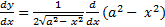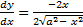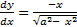Now y=√(a2 -x2
So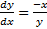x + y(dy/dx) =0
So, the given function is the solution of the corresponding differential equation.

Question 11:
The numbers of arbitrary constants in the general solution of a differential equation of
fourth order are:
(A) 0
(B) 2
(C) 3
(D) 4
Solution
We know that the number of constants in the general solution of a differential equation
of order n is equal to its order.
Therefore, the number of constants in the general equation of fourth order differential
equation is four.
Hence, the correct answer is D.
Question 12:
The numbers of arbitrary constants in the particular solution of a differential equation of
third order are:
(A) 3
(B) 2
(C) 1
(D) 0
Solution
In a particular solution of a differential equation, there are no arbitrary constants.
Hence, the correct answer is D.

Go back to Class 12 Main Page using below links